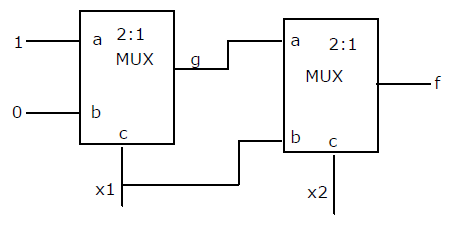Open in App
Not now

# GATE | GATE-CS-2001 | Question 37

• Last Updated : 28 Jun, 2021

Consider the circuit shown below. The output of a 2:1 Mux is given by the function (ac’ + bc).

``````

Which of the following is true?
(A) f = x1’+ x1x
(B) f = x1’x2 + x1x2′
(C) f = x1x2 + x1’x2′
(D) f = x1 + x2′

Explanation:
g = (a and x1′) or (b and x1)
g = (1 and x1’) or (0 and x1)
g = x1’

f = ac’ + bc
f = (a and x2′) or (b and x2)
f = (g and x2′) or (x1 and x2)
f = x1’x2’ + x1x2

Thus, option (C) is the answer.

Please comment below if you find anything wrong in the above post.

Quiz of this Question

My Personal Notes arrow_drop_up